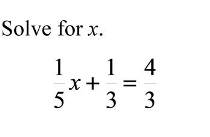# One-step equations

One-step equations are the simplest equations around. Why? Because they take only one step to solve. The main objective is to have only the variable (x or any other letter that is used ) on one side and the numbers on the other side. The number in front of the variable should be the number 1. For example, let us solve this equation:
3 + x = 4
To solve this equation, you need to move the number 3 to the right side of the equation (remeber the „variables on one side, the numbers on the other“). When you do that, it should look like this.
x = 4 – 3
You have probably noticed that the number 3 has changed to -3 when we moved it. When you move numbers or variables from one side to the other, they change signs. Always. It is very important to remember that. The only thing left to do now is to perform the subtraction to get the final result, which is:
x = 1
The other very important thing to remember is that whatever you do to one part of the equation, you have to do with the other as well. We are going to show you on this example:
3x = 9
The only thing you have to do is to get rid of the number 3 in front of the variable x. You can acomplish that by dividing the WHOLE equation with the number 3. Like this:
3x = 9 |:3
x = 1
And now you have a solved equation.
These are the basic rules for solving one-step equations. Hope we helped you learn to solve these simple equations and that you have a good time practicing them using our worksheets.